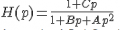# Problem with filter transfer function

#### jn2

Joined Jan 3, 2017
18
Good morning,

I have a problem in understanding the transfer function and the nature of a filter.
The transfer function of the filter is written in the following form, which has the sum of two transfer functions, low pass and band pass.With p = jw; A, B and C are constants.

I would like to know if there is this type of filter that gives the sum of two types of filters on the same terminal ???
If this type of filter does not exist, what is the method for transforming this transfer function to the basic transfer function of the bandpass filter, that is, Neglect the part of the low pass filter ???

#### thumb2

Joined Oct 4, 2015
122
I would like to know if there is this type of filter that gives the sum of two types of filters on the same terminal ???
What makes you think it doesn' t exists ?

BTW you can use LaTeX:

Code:
$$H(s) = \frac{1 + Cs}{As^2 + Bs + 1}$$
$$H(s) = \frac{1 + Cs}{As^2 + Bs + 1}$$

Try to plot the Bode diagram for some value of ABC.

Last edited:

#### jn2

Joined Jan 3, 2017
18
The transfer function of this filter is:
\begin{equation}
H(s)=\frac{1+RCs}{1+RCs+LCs^2}
\end{equation}

Can you tell me the name of this filter and how i can have a bandpass filter from this equation?

#### thumb2

Joined Oct 4, 2015
122
OK. So you can see that the TF is possible.
I don't know the name of the filter and I don't know if it has one, but it reminds me the typical 1st order PLL RC filter which has an R instead of the L.

how i can have a bandpass filter from this equation?
Mmm well, you have to change circuit, of course. You can change from low pass to high pass by simply interchanging the L and C (try with the circuit), but you can't obtain a bandpass from a low pass in that way. You have to add some L or C.

Here you find a table resume of filters transfer functions in therms of the quality factor:
https://en.wikipedia.org/wiki/Q_factor

If you search for LC bandpass filter you will find multiple examples.

Last edited:

#### jn2

Joined Jan 3, 2017
18
Therefore, there is no condition that allows me to neglect the constant term (1) in comparison with the variable term (Cs), in order to have the passband filter.
Moreover, the simulation gives me a passband filter in high frequency as you can notice in the figure of my previous answer.

#### thumb2

Joined Oct 4, 2015
122
Mmm. Wait. I was wrong before in my last post.

jn2 said:
Therefore, there is no condition that allows me to neglect the constant term (1) in comparison with the variable term (Cs), in order to have the passband filter.
Yes, you could just change the circuit to this:the simulation gives me a passband filter in high frequency as you can notice in the figure of my previous answer.
Mmm, no. If you calculate:

\begin{align} \lim_{s \to 0} \left( \frac{1+RCs}{1+RCs+LCs^2}\right ) = 1\\ \lim_{s \to \infty} \left( \frac{1+RCs}{1+RCs+LCs^2}\right ) = 0\\ \end{align}

So this is a low pass filter behaviour. You could also determine it by drawing the low frequency behaviour (L = short circut and C = open circuit) and high frequency behaviour (L = open circuit and C = short circuit).

If we determine the TF of the last circuit we have:

$$H(s) = \frac{R}{\frac{1+RCs+LCs^2}{sC}} = \frac{sRC}{1+RCs+LCs^2}$$

Then:
\begin{align} \lim_{s \to 0} \left(\frac{sRC}{1+RCs+LCs^2} \right ) = 0\\ \lim_{s \to \infty} \left(\frac{sRC}{1+RCs+LCs^2} \right ) = 0\\ \end{align}

this is bandpass behaviour.Last edited:

#### jn2

Joined Jan 3, 2017
18
So, i can say that at the frequency range that interests me (700 MHz for example), my circuit is bandpass filter, with the following TF:
H(s)=\frac{sRC}{1+RCs+LCs^2}

#### thumb2

Joined Oct 4, 2015
122
Yes. But over all the frequency range.